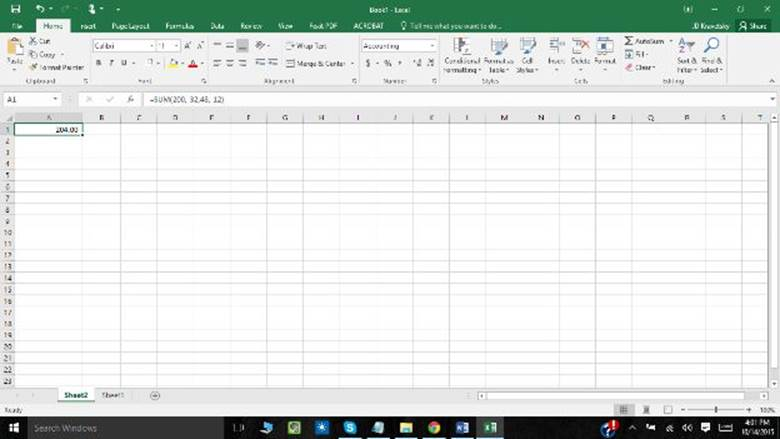﻿ ﻿SUBTRACTING NUMBERS IN EXCEL 2016 FOR WORKBOOKS - Microsoft Office 2016: The Complete Guide (2015)

# Microsoft Office 2016: The Complete Guide (2015)

### SUBTRACTING NUMBERS IN EXCEL 2016 FOR WORKBOOKS.

Please note that selected Excel worksheet functions, calculated results and formula may show slight disparity between a Windows PC using x86 or x86-64 architecture and a Windows RT PC using ARM.

What if you wanted to find out how many inventory items are profitable, that would be calculated by subtracting the total non-profitable items away the numerical value of inventory items. Another example would be calculating the number of employees who are drawing near to retirement age, which would be calculated by subtracting the total number of employees from those who are of age 55. Excel provides more than a few avenues to subtract numbers. This is very resourceful as there are varying arguments in Excel which is used differently by each user. This levels the playing field as now whatever argument you may have to use you can still carry out this function.

Where Can You Subtract Numbers in Excel 2016?

Actually, you can subtract numbers in a cell or in a range.

Subtracting Numbers in a Cell.

The minus sign (=) is the main mathematical operator in a cell. For instance, when the formula

=12-6

Is typed in a cell. The result “6” is given.Subtracting Numbers in a Range

Subtracting numbers in a range differ from subtracting numbers in a cell as the sum function is used. Assigning the negative sign to a number functions the same way as subtracting.

Please be aware of the fact that there is no SUBTRACT function in Excel. Therefore, this method is utilized in carrying out subtraction. For example, =SUM (200, -32,48, -12) produces 204.A more structured example is:

Create a blank workbook or worksheet.

Select the content in cells A1 to B7 (in the following example of a spreadsheet).

P.S. Do not select ANY headers.

3. Press CTRL+C.

4. In the spreadsheet, select cell A1, and then select CTRL+V.

5. In order to switch between viewing the results and viewing the formulas that return the results, press CTRL+ (grave accent) or on the Formulas tab in the

Formula Auditing group, click the Show Formulas Button.

How to use The Formula Function?

The SUM FUNCTION add all the numbers that are given within a specific argument. That argument can vary from being a range, a cell reference, an array, a constant, a formula or the result from another function. One instance can be, SUM (A2:A9), this results in the addition of all numbers that are within the sells A2 to A9(Please note that in this instance “A2:A9” is an example of a range which is an argument. Another example is SUM (A1:A3: A5) adds the numbers contained within cells A1, A3 and A5.

﻿